Next: Tensor Fields Up: Cartesian Tensors Previous: Tensors and Tensor Notation

# Tensor Transformation

As we saw in Appendix A, scalars and vectors are defined according to their transformation properties under rotation of the coordinate axes. In fact, a scalar is invariant under rotation of the coordinate axes. On the other hand, according to Equations (A.49) and (B.6), the components of a general vector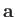transform under an infinitesimal rotation of the coordinate axes according to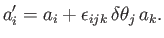(B.24)

Here, the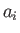are the components of the vector in the original coordinate system, theare the components in the rotated coordinate system, and the latter system is obtained from the former via a combination of an infinitesimal rotation through an angle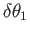about coordinate axis 1, an infinitesimal rotation through an angle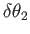about axis 2, and an infinitesimal rotation through an angle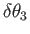about axis 3. These three rotations can take place in any order. Incidentally, a finite rotation can be built up out of a great many infinitesimal rotations, so if a vector transforms properly under an infinitesimal rotation of the coordinate axes then it will also transform properly under a finite rotation.

Equation (B.24) can also be written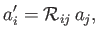(B.25)

where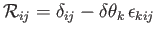(B.26)

is a rotation matrix (which is not a tensor, because it is specific to the two coordinate systems it transforms between). To first order in the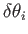, Equation (B.25) can be inverted to give(B.27)

This follows because, to first order in the,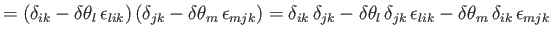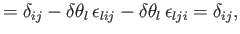(B.28)

where the dummy indexhas been relabeled, and use has been made of Equations (B.8), (B.10), and (B.12). Likewise, it is easily demonstrated that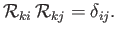(B.29)

It can also be shown that, to first order in the,(B.30)

This follows because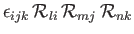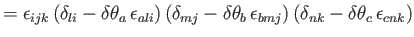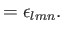(B.31)

Here, there has been much relabeling of dummy indices, and use has been made of Equations (B.10) and (B.16). It can similarly be shown that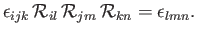(B.32)

As a direct generalization of Equation (B.25), a second-order tensor transforms under rotation as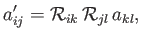(B.33)

whereas a third-order tensor transforms as(B.34)

The generalization to higher-order tensors is straightforward. For the case of a scalar, which is a zeroth-order tensor, the transformation rule is particularly simple: that is,(B.35)

By analogy with Equation (B.27), the inverse transform is exemplified by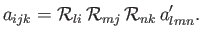(B.36)

Incidentally, because all tensors of the same order transform in the same manner, it immediately follows that two tensors of the same order whose components are equal in one particular Cartesian coordinate system will have their components equal in all coordinate systems that can be obtained from the original system via rotation of the coordinate axes. In other words, if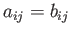(B.37)

in one particular Cartesian coordinate system then(B.38)

in all Cartesian coordinate systems (with the same origin and system of units as the original system). Conversely, it does not make sense to equate tensors of different order, because such an equation would only be valid in one particular coordinate system, and so could not have any physical significance (because the laws of physics are coordinate independent).

It can easily be shown that the outer product of two tensors transforms as a tensor of the appropriate order. Thus, if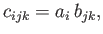(B.39)

and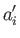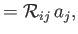(B.40)(B.41)

then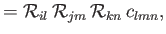(B.42)

which is the correct transformation rule for a third-order tensor.

The tensor transformation rule can be combined with the identity (B.29) to show that the scalar product of two vectors transforms as a scalar. Thus,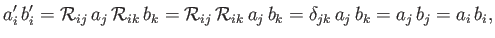(B.43)

where use has been made of Equation (B.14). Again, the previous proof is more rigorous than that given in Section A.6. The proof also indicates that the inner product of two tensors transforms as a tensor of the appropriate order.

The result that both the inner and outer products of two tensors transform as tensors of the appropriate order is known as the product rule. Closely related to this rule is the so-called quotient rule, according to which if (say)(B.44)

where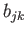is an arbitrary tensor, and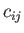transforms as a tensor under all rotations of the coordinate axes, then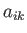--which can be thought of as the quotient ofand--also transforms as a tensor. The proof is as follows: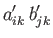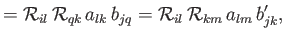(B.45)

where use has been made of the fact thatandtransform as tensors, as well as Equation (B.28). Rearranging, we obtain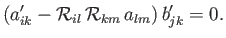(B.46)

However, theare arbitrary, so the previous equation can only be satisfied, in general, if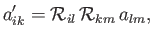(B.47)

which is the correct transformation rule for a tensor. Incidentally, the quotient rule applies to any type of valid tensor product.

The components of the second-order identity tensor,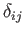, have the special property that they are invariant under rotation of the coordinate axes. This follows because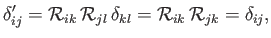(B.48)

where use has been made of Equation (B.28). The components of the third-order permutation tensor,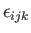, also have this special property. This follows because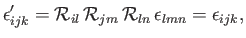(B.49)

where use has been made of Equation (B.31). The fact thattransforms as a proper third-order tensor immediately implies, from the product rule, that the vector product of two vectors transforms as a proper vector: that is,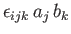is a first-order tensor provided thatand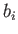are both first-order tensors. This proof is much more rigorous that that given earlier in Section A.8.Next: Tensor Fields Up: Cartesian Tensors Previous: Tensors and Tensor Notation
Richard Fitzpatrick 2016-03-31